## Plotting Fractals with Wolfram Alpha

May 18th, 2009 | Categories: Fractals, Wolfram Alpha | Tags:

I saw a tweet from someone this morning which mentioned that you could plot the Julia Set using Wolfram Alpha.  I had to try this for myself as soon as I could and, sure enough, you can plot the Julia set for any complex number Z: -0.765+0.003 I for example.Very nice but what else can it do.  If I Walpha fractals then I get the following output so I’d expect Wolfram Alpha to compute at least the Koch snow flake, the Sierpinski gasket, the Haferman carpet and the curlicue fractal and, sure enough, it does (click on the links to see for yourself)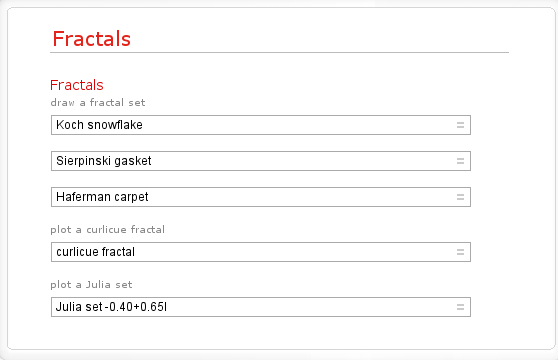Here is a screenshot for the Koch Fractal.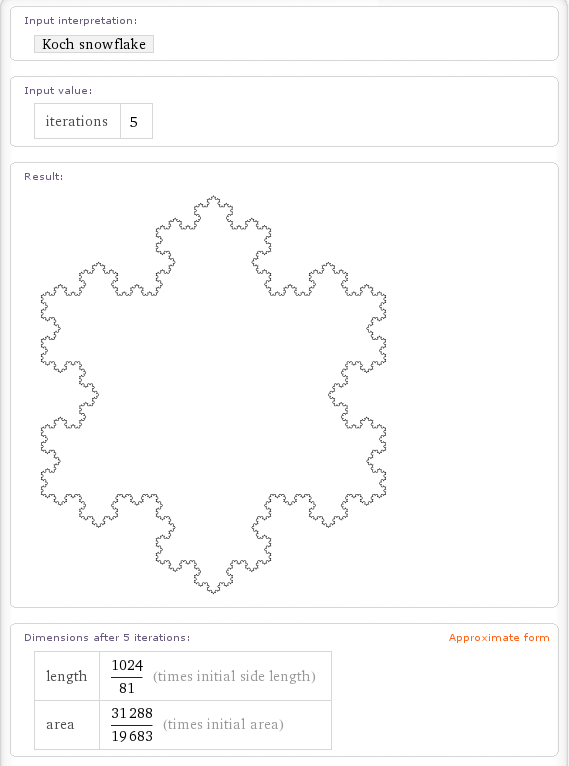These aren’t the only fractals it knows about though. If you walpha Pentaflake (a fractal close to my heart) then you get the following.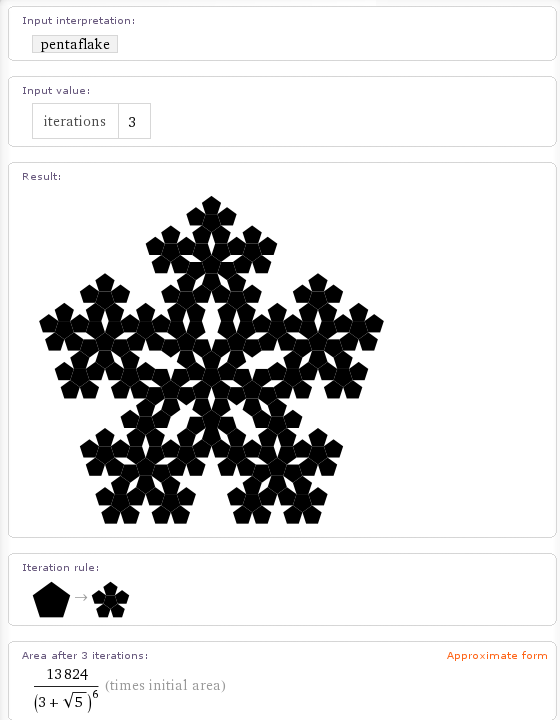Wolfram Alpha can also calculate the H-Fractal.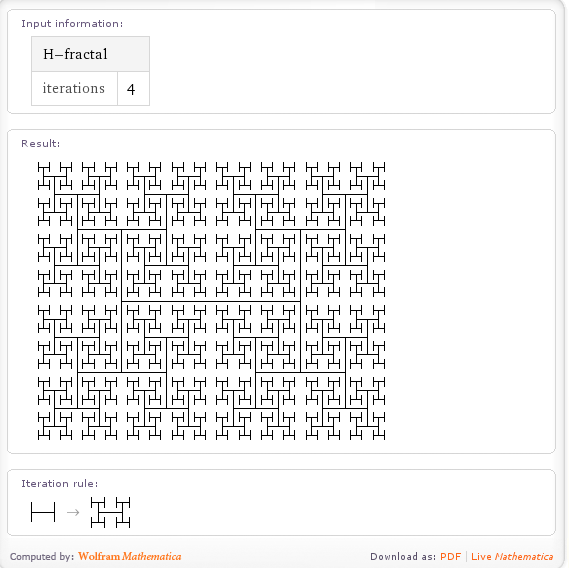Cantor dust is in there too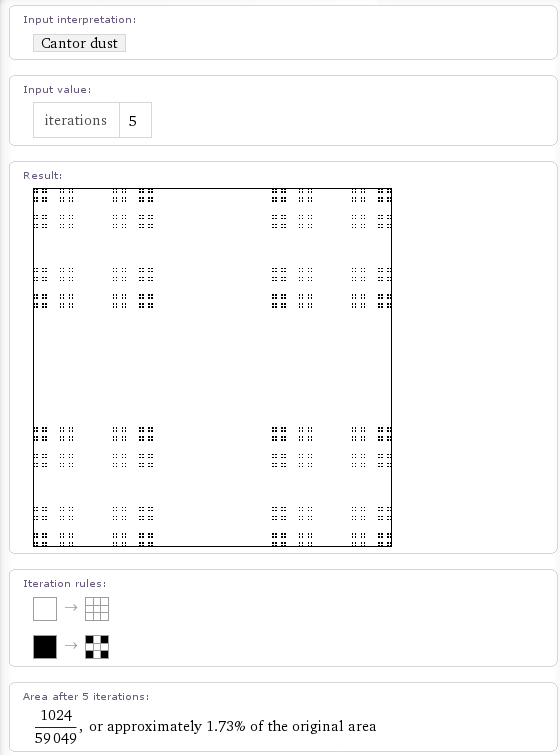as is the box fractal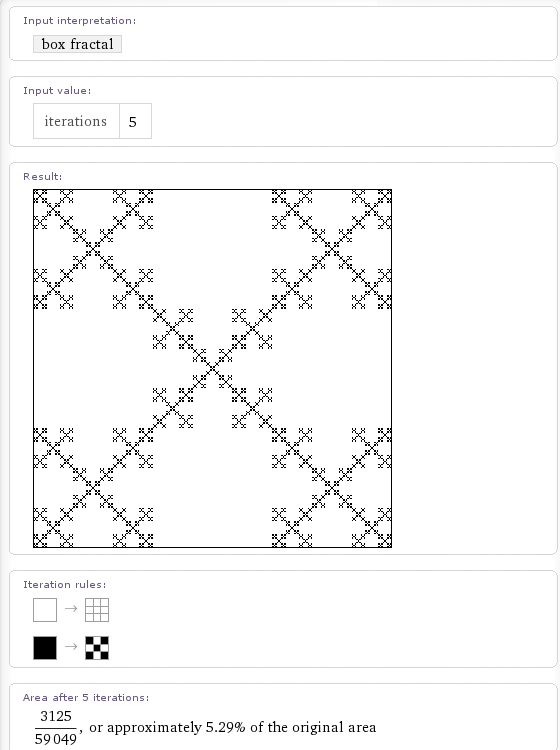It also looks like they are in the middle of implementing the Cesaro fractal.  If you walpha the term then it tries to generate the fractal for a phase angle of pi/3 radians and 5 iterations.  A few seconds later and the calculation times out.  If you lower the number of iterations it returns a red box such as the one below.  If my memory serves, Mathematica returns such a box if there is a problem with the graphics output.  I hope to see this fixed soon.

Update:15th July 2009 – The Cesaro Fractal has now been fixed)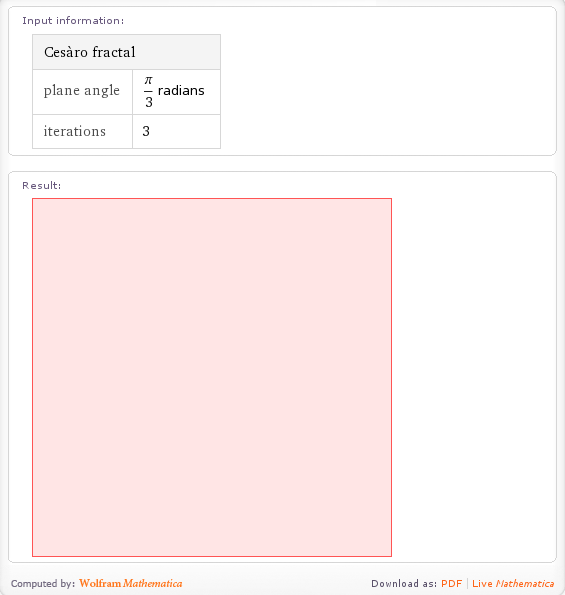It also seems to know about the following fractals but doesn’t seem to calculate anything for them (yet).  I say that it seems to know about them because it gives you an input field for ‘Iterations’ which implies that it knows that a number of iterations makes sense in this context.  It’ll be cool to see all of these implemented in time.

(Note: None of these are implemented yet and may never be – I’ll update if that changes)

• Menger Sponge Update 21st December 2010 – this has now been implemented
• Gosper Island Update:15th July 2009 – this has now been implemented
• Apollonian Gasket Update 21st December 2010 – this has now been implemented
• Dragon Curve Update 21st December 2010 – this has now been implemented
• Hilbert Curve Update 21st December 2010 – this has now been implemented
• Peano curve Update 21st December 2010 – this has now been implemented
• Cantor Set Update:15th July 2009 – this has now been implemented

Some odd omissions (at the time of writing) are the Mandelbrot set (Update: 15th July 2009 – this has been done now) and the Lorenz attractor.

This is all seriously cool stuff for Fractal fans and shows the power of the Wolfram Alpha idea.  Let me know if you discover any more Fractals that it knows about and I’ll add them here.

Update: I’ve found a few more computable fractals in Wolfram Alpha.  Please forgive me for the lack of screenshots but this is getting to be a rather graphic-intensive post.  The links will take you to a Wolfram Alpha query.

1. Neat! I especially like how for many of them it shows you the “rule” used to generate the fractal, implying (as I would expect, given the descriptions of Wolfram Alpha I’ve read) that it knows about several different generic types of fractal and just generates the requested one on the fly from the associated rule — meaning that adding more fractals is only a matter of minutes.

2. i most like Walpha Easter Eggs,:)

3. Wow, that looks pretty nice! Thanks for sharing it with me.